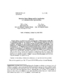## Iteration Space Slicing and Its Application to Communication Optimization##### Files
CS-TR-3737.ps(436.18 KB)
Program slicing is an analysis that answers questions such as Which statements might affect the computation of variable $v$ at statement $s$?'' or Which statements depend on the value of $v$ computed in statement $s$?''. The answers computed by program slicing are generally a set of statements. We introduce the idea of {\em iteration spacing slicing}: we refine program slicing to ask questions such as Which iterations of which statements might effect the computation in iterations $I$ of statement $s$?'' or Which iterations of which statements depend on the value computed by iterations $I$ of statement $s$?''. One application of this general-purpose technique is optimization of interprocessor communication in data-parallel compilers. For example, we can separate a code fragment into 1) those iterations that must be done before a send, 2) those iterations that don't need to be done before a send and don't depend on non-local data and 3), those iterations that depend on non-local data. We examine applications of iteration space slicing to communication optimizations in parallel executions of programs such as stencil computations and block-cyclic Gaussian elimination with partial pivoting. (Also cross-referenced as UMIACS-TR-97-02)